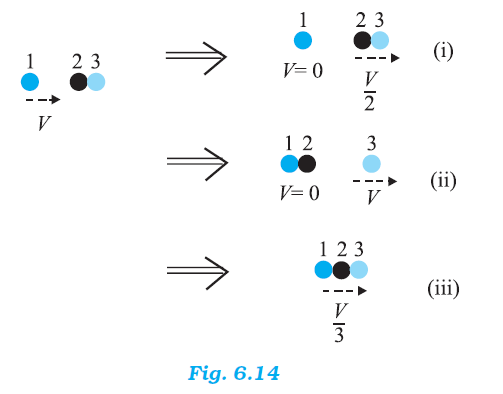## Pages

### Work, Energy and Power NCERT Solutions Class 11 Physics - Solved Exercise Question 6.16

Question 6.16:
Two identical ball bearings in contact with each other and resting on a frictionless table are hit head-on by another ball bearing of the same mass moving initially with a speed V. If the collision is elastic, which of the following figure is a possible result after collision?Solution:
It can be observed that the total momentum before and after collision in each case is constant.
For an elastic collision, the total kinetic energy of a system remains conserved before and after collision.
For mass of each ball bearing m, we can write:
Total kinetic energy of the system before collision:
= (1/2)mV2 + (1/2)(2m) X 02
= (1/2)mV2

Case (i)
Total kinetic energy of the system after collision:
= (1/2) m X 0 + (1/2) (2m) (V/2)2
= (1/4)mV2
Hence, the kinetic energy of the system is not conserved in case (i).

Case (ii)
Total kinetic energy of the system after collision:
= (1/2)(2m) X 0 + (1/2)mV2
= (1/2) mV2
Hence, the kinetic energy of the system is conserved in case (ii).

Case (iii)
Total kinetic energy of the system after collision:
= (1/2)(3m)(V/3)2
= (1/6)mV2
Hence, the kinetic energy of the system is not conserved in case (iii).